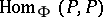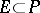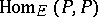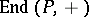# Jacobson-Bourbaki theorem

(diff) ← Older revision | Latest revision (diff) | Newer revision → (diff)
Letbe a finite group of automorphisms of a field, and letbe the subfield of elements ofthat are invariant under the action of. Thenis a normal and separable extension of(cf. Extension of a field), and there is a one-to-one correspondence between the subfields ofcontainingand the subgroups of(cf. also Galois theory). The elements ofare linear operators on the vector spaceover; by the operation of multiplication, the elements ofcan be represented as linear operators onover(the regular representation), and the ringof all linear operators onoveris generated byand; indeed, it is the cross product ofand.
Now, letbe a subfield ofsuch thatis a finite-dimensional vector space overand to each subfieldcontaininglet correspond the ringof linear operators on the vector spaceover. The Jacobson–Bourbaki theorem asserts that this correspondence is a bijection between the subfields ofcontainingand the set of subrings ofthat are left vector spaces overof finite dimension. Moreover, the dimension ofoverequals the dimension ofover.
The Jacobson–Bourbaki theorem can be regarded as an instance of Morita duality. From the theory of Morita equivalence the following very general result is obtained. Letbe a ring, letbe the ring of endomorphisms of the additive group ofan note thatis a left module over. There is a one-to-one correspondence between those subringsofsuch thatis a finitely generated projective generator in the category of right-modules and the subringssuch thatis a submodule of the left-moduleandis a finitely generated projective generator in the category of left-modules.